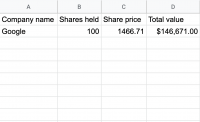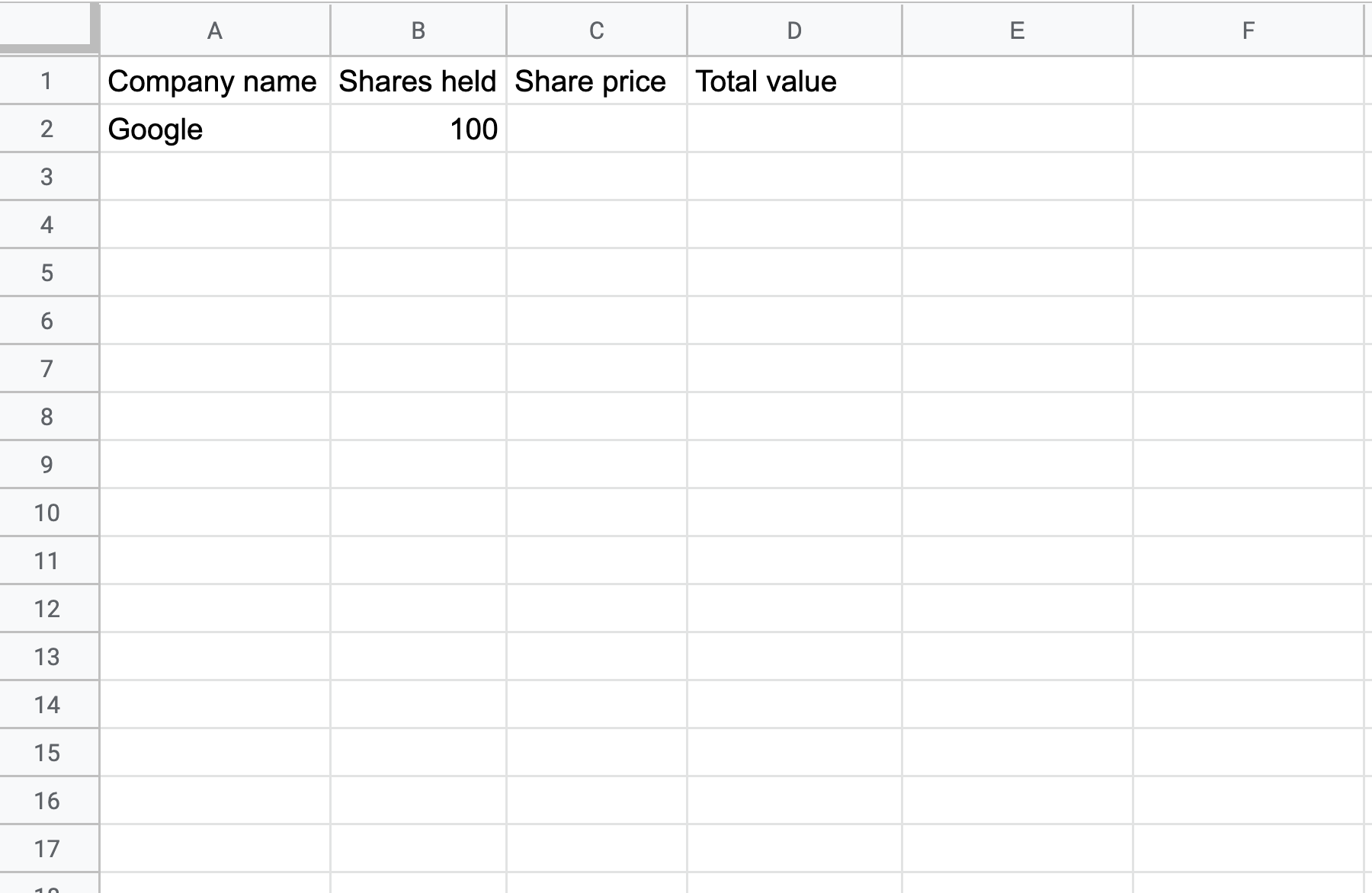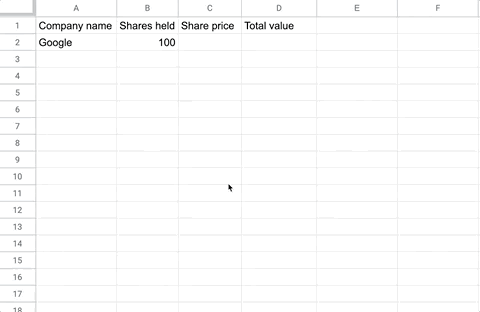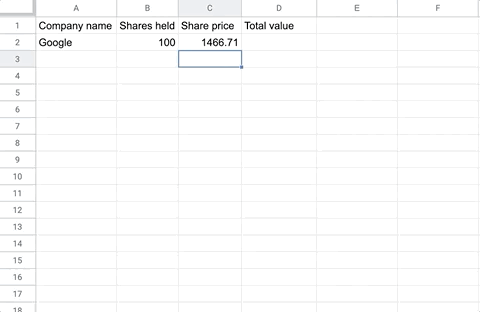# How to track stock prices in Google sheetsTutorial
Jan 26 2020

You can now use the GOOGLEFINANCE function to track stock prices in real time. Using this function you can easily keep track of your stock portfolio value using live data. The GOOGLE finance function is particularly useful when you need customised calculations involving stock prices.

In this tutorial we will use the GOOGLEFINANCE function to lookup the live share value for Google, and calculate the portfolio value based on the number of shared held.

## Function syntax

``=GOOGLEFINANCE(ticker, [attribute], [start_date], [end_date|number_of_days], [interval])``

## Examples

``=GOOGLEFINANCE("GOOG")``

## Step 1: Prepare your dataA - Share price
B - Shares held
C - Share price
D - Total value

## Step 2: Use the GOOGLEFINANCE function to get the share prices of your sharesIn cell B2, enter the following formula

``=GOOGLEFINANCE("GOOG")``

Then add the formula for the other stock prices lookup it's company name in Google.

## Step 3: Calculate the total valueIn D2 enter the formula

``=B2*C2``

This multiplies the share value by the number of shares held to give the value of the shares held.